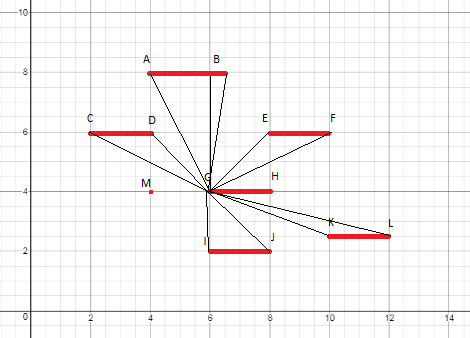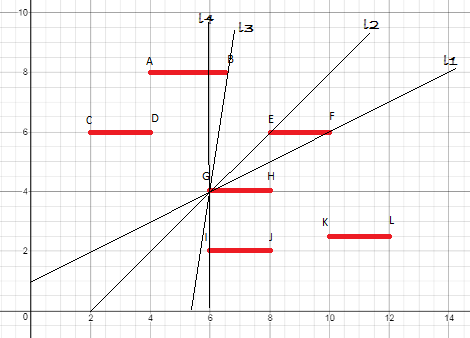# CR192 - Editorial

Author: Saurabh Kumar
Editorialist: Saurabh Kumar

Medium

Line-sweep

### Problem:

We are given the left coordinate and the length of N horizontal lines. We have to draw another line (say L) such that the the sum of length of lines intersected by L is maximized while intersecting minimum number of lines.

### Quick Explanation:

This is a geometry problem which can be solved using the rotating line sweep algorithm. We iterate through all the N lines (targets) and for each end of that line we calculate and store the angle (slope) made by current line and the endpoints of the other N-1 lines (targets). And we sort these values according to the slope. Now we iterate through the values in slope order. If we encounter a slope value corresponding to the first end of some line then we add that length to our current value and on encountering the second end of some line we subtract the length of that line from our current value. We perform the same operation for all the 2 endpoints of the N given lines and calculate the maximum among all the values.

### Explanation:

The basic idea of line-sweep is that we take a line and sweep it in the plane to encounter all the other points present in that plane. As given in the question all the targets are in a 2D plane, therefore, we can use a line to sweep across all the other points (a line segment can be represented using the two end points).

We use rotating line-sweep algorithm in this question. The basic idea is to chose a point P, draw a line passing through that point and then rotate the line about that point. The line extends in both the direction of the point, i.e., the chosen point lies somewhere in the middle of the line. And we rotate that line between angle 0 to 180 degrees and as the line extends in both direction, we cover complete 360 degrees.

Now, some of you must be wondering that there can be infinite lines passing through a fixed point, how can we check for all the lines. The solution is that we do not need to check for all the lines but only the lines formed by the fixed point and the endpoints of the other remaining lines. Once we have calculated and stored the all the angles, we sort them in increasing order. And as we iterate through all the angles, if that angle is formed by the first end of the target we add the length of that target to our currentAnswer and if the angle is formed by the second endpoint then we subtract the length of the line from our currentAnswer. Our finalAnswer if the maximum of all the currentAnswer.Pic1

Now, let us take the above image as our example and try to visualise the algorithm. In that image AB, CD, EF, GH, IJ and KL are our targets. Let the point G be our fixed point about which we are drawing the lines. The different angles calculated are FGH, EGH, BGH and so on for the lines with y co-ordinate greater than G and for the points whose y co-ordinate is less than G, we calculate the angle MGI, MGJ, MGK and so on. After calculating all these angles we store it in some data structure and sort it based on the angles.

Once we have stored the angles and sorted it, we are ready to draw a line and rotate it about the fixed point. We start iterating through the stored angles one by one.Pic2

According to the image the smallest angle formed would be the angle FGH. We see that this angle corresponds to the first endpoint of that line EF therefore, we add the length of that to our variable currentAnswer. Next smallest angle is EGH, and this is formed by the second endpoint of the line EF therefore we subtract the length of EF from currentAnswer. Next comes the angle BGH corresponding to the first endpoint of AB and thus length AB is added to currentAnswer and the next smallest angle is MGI again corresponding to the first endpoint thus the length of line segment IJ is added to currentAnswer and we keep on going like this.

According to the question we need to output the maximum sum of the length of lines intersected and also the number of lines intersected. To acheive this we need a data structure to store the angle, the length of the line and also the count of the line.

``````vector<double, pair<int, int> >
``````

When we calculate the angle from the first endpoint of some line of length L, we push the angle, +L and +1 in the vector and when calculating from the second end point we push back angle, -L and -1 into the vector.

``````if(y < Y[i])
{
v.push_back(make_pair(atan2(Y[i] - y, X1[i] - x), make_pair(-1, -L)));
v.push_back(make_pair(atan2(Y[i] - y, X2[i] - x), make_pair(1, L)));
}
else if(y > Y[i])
{
v.push_back(make_pair(atan2(y - Y[i], x - X1[i]), make_pair(1, L)));
v.push_back(make_pair(atan2(y - Y[i], x - X2[i]), make_pair(-1, -L)));
}
``````

In the above code (X1[i], Y[i]) correspond to the left end of the line segment and (X2[i], Y[i]) corresponds to the right endpoint of the line.

``````sort(v.begin(), v.end());
for(int i=0; i<v.size(); i++)
{
cur = cur + v[i].second.second;
curT = curT + v[i].second.first;
if(cur > ans)
{
ans = cur;
targets = curT;
}
else if(cur == ans)
{
targets = min(targets, curT);
}
}
``````

And in this way we calculate the answer.
The above algorithm takes O(N*log(N)) time for storing all the angles and sorting them. Now, we have to perform this same operation for both the endpoints of all the N line segments.

Time Complexity of our solution becomes O(N*N*log(N)).

Author’s solution can be found here.

Feel free to give your suggestions and feedbacks, they are always welcome.One other similar question you can try.